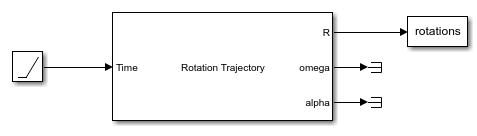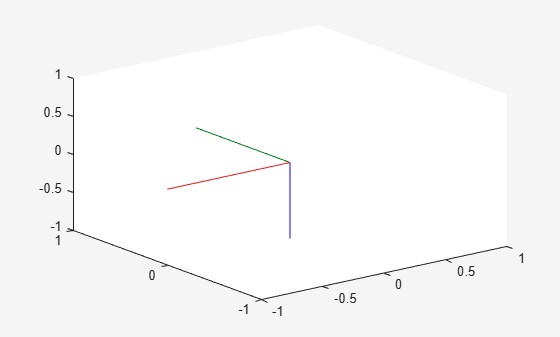# Generate Rotation Trajectory

This example shows how to generate a trajectory that interpolates between rotations using the Rotation Trajectory block.

Open and simulate the model. The Rotation Trajectory block outputs the trajectory between two rotations and saves the intermediate rotations to the `rotations` variable. This example generates a simple rotation trajectory from the x-axis to the z-axis.

```open_system('rot_traj_ex1.slx') simOut = sim('rot_traj_ex1.slx');```Use `plotTransforms` to plot the rotation trajectory.

```numRotations = size(simOut.rotations,3); translations = zeros(3,numRotations); figure("Visible","on") for i = 1:numRotations plotTransforms(translations(:,i)',simOut.rotations(:,i)') xlim([-1 1]) ylim([-1 1]) zlim([-1 1]) drawnow pause(0.1) end```